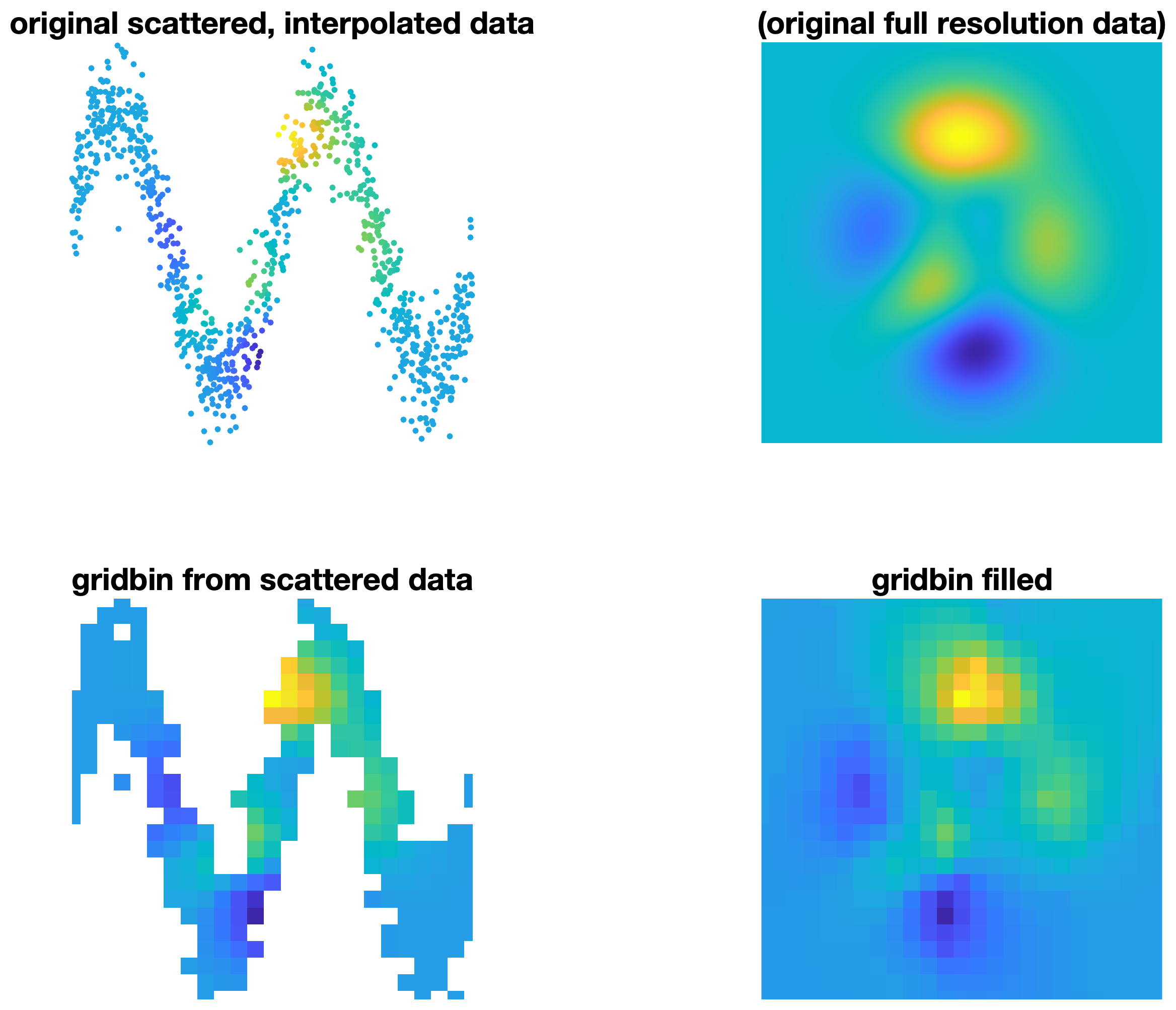gridbin

version 1.0.0 (533 KB) by
A MATLAB function for gridding large datasets of scattered data.

Updated 26 Dec 2021

From GitHubgridbin

A fast MATLAB function for gridding very large datasets of scattered data. This function is somewhat similar to Matlab's built-in griddata or John D'Errico's gridfit, with the differences being that

1. gridbin returns the mean value of all scattered data points within every cell of a grid rather than interpolating or fitting a surface.
2. gridbin is much faster than griddata or gridfit.
3. gridbin can handle much larger scattered datasets than griddata or gridfit.
4. gridbin can return statistics. For example, gridbin can return the standard deviation of all scattered data points within a grid cell simply by adding @std to the function call.

Syntax

vq = gridbin(x,y,v,xq,yq)
vq = gridbin(...,@func)
[vq,N] = gridbin(...)

Description

vq = gridbin(x,y,v,xq,yq) produces a 2D grid of values vq at the coordinates xq,yq. Inputs x,y,v may be scattered data, and the output vq is the mean of all scattered values v within each spatial bin.

vq = gridbin(...,@func) applies any function @func to the v data. By default, @func is @mean, meaning that vq contains the mean of all values v within each spatial bin.

[vq,N] = gridbin(...) also returns a grid N containing the number of observations v in each bin.Cite As

MATLAB Release Compatibility
Created with R2021a
Compatible with any release
Platform Compatibility
Windows macOS Linux